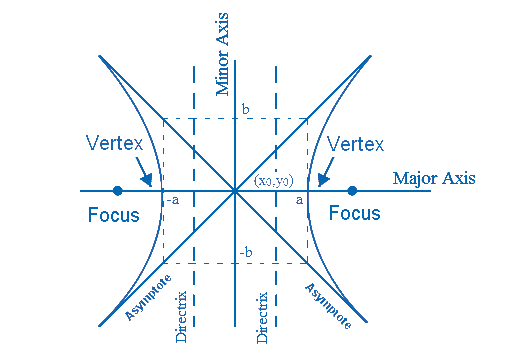# HYPERBOLA FORMULA

In simple sense, hyperbola looks similar to to mirrored parabolas. The two halves are called the branches. When the plane intersect on the halves  of a right circular cone angle of which will be parallel to the axis of the cone, a parabola is formed.

A hyperbola contains: two foci and two vertices. The foci of the hyperbola are away from the hyperbola’s center and vertices. Here is an illustration to make you understand:The equation for hyperbola is,

$\large \frac{(x-x_{0})^{2}}{a^{2}}-\frac{(y-y_{0})^{2}}{b^{2}}=1$

Where,

$$\begin{array}{l}x_{0}, y_{0}\end{array}$$
are the center points.
$$\begin{array}{l}a\end{array}$$
= semi-major axis.
$$\begin{array}{l}b\end{array}$$
= semi-minor axis.

Let us learn the basic terminologies related to hyperbola formula:

#### MAJOR AXIS

The line that passes through the center, focus of the hyperbola and vertices is the Major Axis. Length of the major axis = 2a. The equation is given as:

$\large y=y_{0}$

#### MINOR AXIS

The line perpendicular to the major axis and passes by the middle of the hyperbola is the Minor Axis.
Length of the minor axis = 2b. The equation is given as:

$\large x=x_{0}$

#### ECCENTRICITY

The variation in the conic section being completely circular is eccentricity. It is usually greater than 1 for hyperbola. Eccentricity is

$$\begin{array}{l}2\sqrt{2}\end{array}$$
for a regular hyperbola. The formula for eccentricity is:

$\large \frac{\sqrt{a^{2}+b^{2}}}{a}$

#### ASYMPTOTES

Two bisecting lines that is passing by the center of the hyperbola that doesn’t touch the curve is known as the Asymptotes. The equation is given as:

$\large y=y_{0}+\frac{b}{a}x-\frac{b}{a}x_{0}$

$\large y=y_{0}-\frac{b}{a}x+\frac{b}{a}x_{0}$

#### Directrix of a hyperbola

Directrix of a hyperbola is a straight line that is used in generating a curve. It can also be defined as the line from which the hyperbola curves away from. This line is perpendicular to the axis of symmetry. The equation of directrix is:

$\large x=\frac{\pm a^{2}}{\sqrt{a^{2}+b^{2}}}$

#### VERTEX

The point of the branch that is stretched and is closest to the center is the vertex. The vertex points are

$$\begin{array}{l}\LARGE \left(a,y_{0}\right ) \ and \ \LARGE \left(-a,y_{0}\right )\end{array}$$

#### Focus (foci)

On a hyperbola, focus (foci being plural) are the fixed points such that the difference between the distances are always found to be constant. The two focal points are:

$\large\left(x_{0}+\sqrt{a^{2}+b^{2}},y_{0}\right)$

$\large \left(x_{0}-\sqrt{a^{2}+b^{2}},y_{0}\right)$

### Solved examples

Question: The equation of the hyperbola is given as:

$$\begin{array}{l}\frac{(x-4)^{2}}{9^{2}}-\frac{(y-2)^{2}}{7^{2}}\end{array}$$

Find the following: Vertex, Asymptote, Major Axis, Minor Axis and Directrix?

Solution:

Given,

$$\begin{array}{l}x_{0}=4\end{array}$$

$$\begin{array}{l}y_{0}=2\end{array}$$

$$\begin{array}{l}a =9\end{array}$$

$$\begin{array}{l}b = 7\end{array}$$

The vertex point:

$$\begin{array}{l}(a, y_{0})\end{array}$$
and
$$\begin{array}{l}(-a, y_{0})\end{array}$$
are
$$\begin{array}{l}(9, 2)\end{array}$$
and
$$\begin{array}{l}(-9, 2)\end{array}$$

Asymptote

$$\begin{array}{l}y=\frac{7}{9}(x-4)+2\\ y=-\frac{7}{9}(x-4)+2\end{array}$$

Major Axis

a = 9

Minor Axis

b = 7

Directrix

$$\begin{array}{l}x=\frac{\pm 9^{2}}{\sqrt{9^{2}+7^{2}}} = \pm \frac{81}{\sqrt{81+49}}=7.1\end{array}$$